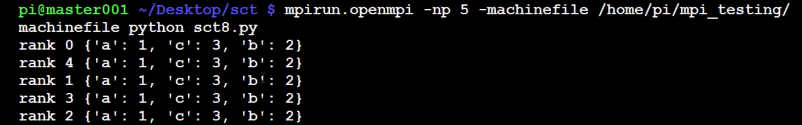MPI broadcasting tutorial with Python, mpi4py, and bcast

MPI bcast example in Python

```from mpi4py import MPI

comm = MPI.COMM_WORLD
rank = comm.rank

if rank == 0:
data = {'a':1,'b':2,'c':3}
else:
data = None

data = comm.bcast(data, root=0)
print 'rank',rank,data
```

What's happening is, first, we assign some data to rank 0, the master node. Then, we want to "broadcast" with bcast the data to all of the other nodes. We are then setting all data to None first, so all other nodes have None as their data.

Next, we use comm.bcast() to broadcast the data from rank 0. So, the first parameter is the data, the second, "root," means "from what node." With broadcast, all nodes automatically accept the data, under the data var name.

`mpirun.openmpi -np 5 -machinefile /home/pi/mpi_testing/machinefile python ~/Desktop/sct/sct8.py`The next tutorial:• Build a Supercomputer with Raspberry Pis

• Intro

• Supplies

• Installing Operating System

• Testing Supercomputer

• MPI with MPI4py Introduction

• Installing mpi4py for use with Python and MPI

• First basic MPI script with mpi4py

• Using conditional, Python, statements alongside MPI commands example

• Getting network processor size with the size command

• Sending and Receiving data using send and recv commands with MPI

• Dynamically sending messages to and from processors with MPI and mpi4py

• Message and data tagging for send and recv MPI commands tutorial

• MPI broadcasting tutorial with Python, mpi4py, and bcast
• Scatter with MPI tutorial with mpi4py

• Gather command with MPI, mpi4py, and Python